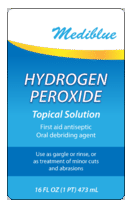# 7.17: Percent Solutions

$$\newcommand{\vecs}{\overset { \rightharpoonup} {\mathbf{#1}} }$$ $$\newcommand{\vecd}{\overset{-\!-\!\rightharpoonup}{\vphantom{a}\smash {#1}}}$$$$\newcommand{\id}{\mathrm{id}}$$ $$\newcommand{\Span}{\mathrm{span}}$$ $$\newcommand{\kernel}{\mathrm{null}\,}$$ $$\newcommand{\range}{\mathrm{range}\,}$$ $$\newcommand{\RealPart}{\mathrm{Re}}$$ $$\newcommand{\ImaginaryPart}{\mathrm{Im}}$$ $$\newcommand{\Argument}{\mathrm{Arg}}$$ $$\newcommand{\norm}{\| #1 \|}$$ $$\newcommand{\inner}{\langle #1, #2 \rangle}$$ $$\newcommand{\Span}{\mathrm{span}}$$ $$\newcommand{\id}{\mathrm{id}}$$ $$\newcommand{\Span}{\mathrm{span}}$$ $$\newcommand{\kernel}{\mathrm{null}\,}$$ $$\newcommand{\range}{\mathrm{range}\,}$$ $$\newcommand{\RealPart}{\mathrm{Re}}$$ $$\newcommand{\ImaginaryPart}{\mathrm{Im}}$$ $$\newcommand{\Argument}{\mathrm{Arg}}$$ $$\newcommand{\norm}{\| #1 \|}$$ $$\newcommand{\inner}{\langle #1, #2 \rangle}$$ $$\newcommand{\Span}{\mathrm{span}}$$$$\newcommand{\AA}{\unicode[.8,0]{x212B}}$$

There are human cultures that do not recognize numbers above three. Anything greater than that is simply referred to as "much" or "many". Although this form of calculation may seem very limited, American culture does the same thing to a certain degree. For example, there are several ways to express the amount of solute in a solution in a quantitative manner. The concentration of a solution is a measure of the amount of solute that has been dissolved in a given amount of solvent or solution. A concentrated solution is one that has a relatively large amount of dissolved solute. A dilute solution is one that has a relatively small amount of dissolved solute. However, those terms are vague, and it is often necessary to express concentration with numbers.

## Percent Solutions

One way to describe the concentration of a solution is by the percent of a solute in the solvent. The percent can further be determined in one of two ways: (1) the ratio of the mass of the solute divided by the mass of the solution or (2) the ratio of the volume of the solute divided by the volume of the solution.

### Mass Percent

When the solute in a solution is a solid, a convenient way to express the concentration is by mass percent $$\left( \frac{\text{mass}}{\text{mass}} \right)$$, which is the grams of solute per $$100 \: \text{g}$$ of solution.

$\text{Percent by mass} = \frac{\text{mass of solute}}{\text{mass of solution}} \times 100\%\nonumber$

Suppose that a solution was prepared by dissolving $$25.0 \: \text{g}$$ of sugar into $$100 \: \text{g}$$ of water. The percent by mass would be calculated by:

$\text{Percent by mass} = \frac{25 \: \text{g sugar}}{125 \: \text{g solution}} \times 100\% = 20\% \: \text{sugar}\nonumber$

Sometimes you may want to make up a particular mass of solution of a given percent by mass, and need to calculate what mass of the solvent to use. For example, you need to make $$3000 \: \text{g}$$ of a $$5\%$$ solution of sodium chloride. You can rearrange and solve for the mass of solute:

$\text{mass of solute} = \frac{\text{percent by mass}}{100\%} \times \text{mass of solution} = \frac{5\%}{100\%} \times 3000 \: \text{g} = 150 \: \text{g} \: \ce{NaCl}\nonumber$

You would need to weigh out $$150 \: \text{g}$$ of $$\ce{NaCl}$$ and add it to $$2850 \: \text{g}$$ of water. Notice that it was necessary to subtract the mass of the $$\ce{NaCl}$$ $$\left( 150 \: \text{g} \right)$$ from the mass of solution $$\left( 3000 \: \text{g} \right)$$ to calculate the mass of the water that would need to be added.

### Volume Percent

The percentage of solute in a solution can more easily be determined by volume when the solute and solvent are both liquids. The volume of the solute divided by the volume of the solution, expressed as a percent, yields the percent by volume $$\left( \frac{\text{volume}}{\text{volume}} \right)$$ of the solution. If a solution is made by adding $$40 \: \text{mL}$$ of ethanol to $$20 \: \text{mL}$$ of water, the percent by volume is:

\begin{align*} \text{Percent by volume} &= \frac{\text{volume of solute}}{\text{volume of solution}} \times 100\% \\ &= \frac{40 \: \text{mL ethanol}}{240 \: \text{mL solution}} \times 100\% \\ &= 16.7\% \: \text{ethanol} \end{align*}\nonumber

Frequently, ingredient labels on food products and medicines have amounts listed as percentages (see figure below).Figure $$\PageIndex{1}$$: Hydrogen peroxide is commonly labeled as a $$3\%$$ by volume solution for use as a disinfectant.

## Summary

• The concentration of a solution is a measure of the amount of solute that has been dissolved in a given amount of solvent or solution.
• A concentrated solution has a relatively large amount of dissolved solute.
• A dilute solution has a relatively small amount of dissolved solute.
• Techniques for calculation of percent mass and percent volume solution concentrations are described.

7.17: Percent Solutions is shared under a CC BY-NC license and was authored, remixed, and/or curated by LibreTexts.